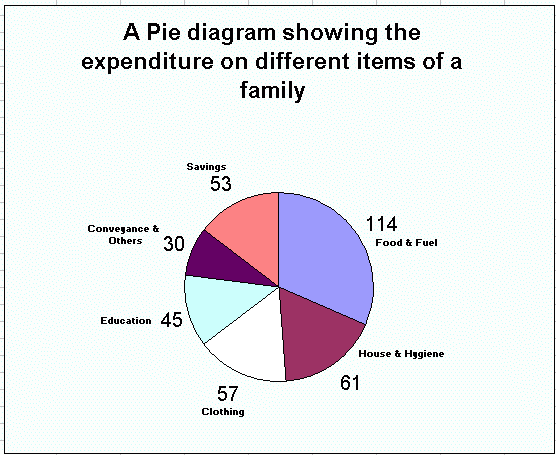Email us to get an instant 20% discount on highly effective K-12 Math & English kwizNET Programs!

#### Online Quiz (WorksheetABCD)

Questions Per Quiz = 2 4 6 8 10

### Grade 7 - Mathematics10.9 Pie Diagram

Steps:

1. Take the total value of items equal to 360 degrees.

2. Convert all components of the data into degrees using the formula,
Degrees of any component part = (Component value/total value) * 360 degrees.

3. Draw a circle of appropriate radius.

4. Draw any radius preferable horizontal, if the circle, with this radius as base line draw an angle at the centre equal to the degree represented to the first component. This angular ray will tough the circumference of the circle. This sector so obtained with represent the proportion of the first component. Taking the second line as a base draw an angle equal to the degree represented by the second component and so on till all the components are completed.

5. Write the title of the diagram.

Example:
Draw a pie diagram for the given data.
Item of expenditureFood & FuelHouse & HygieneClothingEducationConveyance & OthersSavings
Amount Spent in Dollars1500800750600400700

Solution:
Total income of the family = \$4,750
Calculation for the diagram
Food & Fuel = \$1500 = (1500/4750) * 360 = 114 degrees (Approximately)
House & Hygiene = \$800 = (800/4750) */ 360 = 61 degrees (Approximately)
Clothing = \$750 = (750/4750) * 360 = 57 degrees (Approximately)
Education = \$600 = (600/4750) * 360 = 45 degrees (Approximately)
Conveyance & Others = \$400 = (400/4750) * 360 = 30 degrees (Approximately)
Saving = \$700 = (700/4750) * 360 = 53 degrees (Approximately)Directions: Draw the pie diagram for the following data.

1. Draw a pie diagram for the given data.
Item of expenditureFood & FuelHouse & HygieneClothingEducationConveyance & OthersSavings
Amount Spent in Dollars16001800950500800800

2. Draw a pie diagram for the given data.
Item of expenditureFood & FuelHouse & HygieneClothingEducationConveyance & OthersSavings
Amount Spent in Dollars140016001250150015001800

3. Draw a pie diagram for the given data.
Item of expenditureFood & FuelHouse & HygieneClothingEducationConveyance & OthersSavings
Amount Spent in Dollars24001300155025003500800